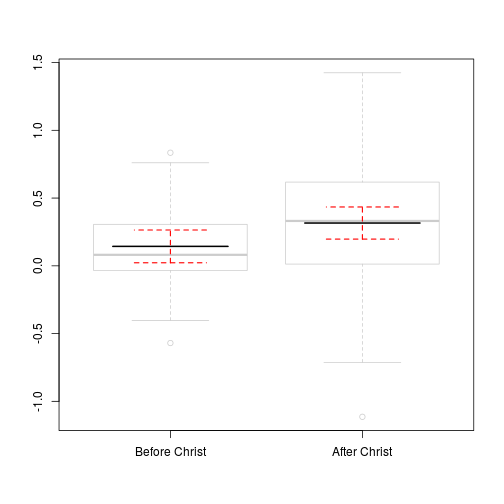## Paul Johnson 20130520, 20140805
## Investigates tricky problem with addition of regression lines
## to box plots.

## Note: I think a "line" is a wrong way to plot the effect of
## a categorical predictor.  But this is a pretty interesting
## example of the way factors in R can produce surprising
## results.

## There are more-or-less good "automatic" ways to do this in various
## R packages.  You could try the termplot function in the base R
## stats package. This work is partly intended to help users
## understand the basic idea of a factor variable and how R tools
## characterize and interact with them.

set.seed(234234)
x <- sample(c("AD","BC"), 100, replace = TRUE)
xf <- factor(x, levels = c("BC", "AD"),
labels = c("Before Christ","After Christ"))
##y <- rnorm(100) + contrasts(xf)[xf] * 0.5
y <-  rnorm(100, mean = as.numeric(xf) * 0.15,
sd = as.numeric(xf) * 0.3)
dat <- data.frame(x, xf, y)
rm(x, xf, y) ## tidiness is next to godliness

m1 <- lm (y ~ xf, data = dat)

plot(y ~ xf, data = dat)

abline (m1)## Just a little problem the line does not "go through" the box
## plot in the right spot because contrasts(xf) is 0,1 but
## the plot uses xf in 1,2.

xlevels <- levels(dat$xf) newdf <- data.frame(xf = xlevels) newdf$fit <- predict(m1, newdata = newdf)
newdf

##              xf    fit
## 1 Before Christ 0.1435
## 2  After Christ 0.3158

##Watch now: the plot comes out "reversed", AC before BC
plot(y ~ xf, data = dat)
lines(fit ~ xf, newdf)## Ach. That's horrible.

## Its a puzzler. Better look at the regression

summary(m1)

##
## Call:
## lm(formula = y ~ xf, data = dat)
##
## Residuals:
##     Min      1Q  Median      3Q     Max
## -1.4297 -0.2181 -0.0387  0.2590  1.1089
##
## Coefficients:
##                Estimate Std. Error t value Pr(>|t|)
## (Intercept)      0.1435     0.0609    2.36    0.020 *
## xfAfter Christ   0.1723     0.0853    2.02    0.046 *
## ---
## Signif. codes:  0 '***' 0.001 '**' 0.01 '*' 0.05 '.' 0.1 ' ' 1
##
## Residual standard error: 0.426 on 98 degrees of freedom
## Multiple R-squared:  0.04,   Adjusted R-squared:  0.0302
## F-statistic: 4.08 on 1 and 98 DF,  p-value: 0.0461

## Ah. Now I see. Compare

levels(dat$xf)  ##  "Before Christ" "After Christ"  levels(newdf$xf)

##  "After Christ"  "Before Christ"

## Why doesnt newdf$xf respect the ordering of the levels? xlevels  ##  "Before Christ" "After Christ"  ## This seems a little horrible to me. newdf$xf was
## created "afresh", and it alphabetized the levels. Hassle

newdf$xf <- relevel(newdf$xf, ref = "Before Christ")
newdf$fit <- predict(m1, newdata = newdf) plot(y ~ xf, data = dat) lines(fit ~ xf, newdf, col = "red", lwd = 2)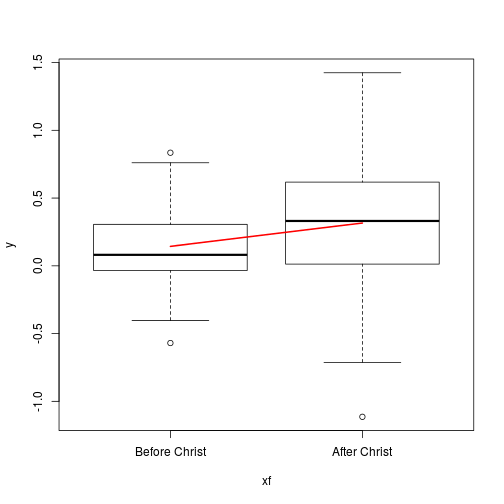## OK, that got it going in the right direction. ## And, since I made y gamma distributed, you now clearly ## see that the fitted (expected) value of y ## is not the same as the observed median. ## ## But did I want that line to stop that way? I want it to ## go a little further. But to decide how, I need to learn ## the coordinate system. ## Lets explore how R is drawing that plot. Recall ## plot is a generic function, R is sending the work to boxplot ## ## This produces the same graph boxplot(y ~ xf, data = dat) ## OK, now realize that boxplot generates an output bp1 <- boxplot(y ~ xf, data = dat) bp1  ##$stats
##          [,1]     [,2]
## [1,] -0.40328 -0.71231
## [2,] -0.03460  0.01279
## [3,]  0.08111  0.33094
## [4,]  0.30614  0.61798
## [5,]  0.76046  1.42464
##
## $n ##  49 51 ## ##$conf
##          [,1]   [,2]
## [1,] 0.004195 0.1970
## [2,] 0.158015 0.4648
##
## $out ##  0.8349 -0.5696 -1.1139 ## ##$group
##  1 1 2
##
## $names ##  "Before Christ" "After Christ"  ## Lets test placement of points: text(0.5, -0.5, "Low Left", pos = 4) text(1.5, -0.5, "Low Mid", pos = 1) text(2.5, -0.5, "Low Right", pos = 2)## It appears I want a line that goes from 0.5 to 2.5 on the x ## axis. xnew <- c(0.5, 2.5) ## We know the line should go through x <- c(1, 2) ## numeric values of xf y <- newdf$fit

## OMG. This is the first time I've ever needed the
## "point-to-point" formula for a linear equation.
## y = y1 + [(y2 - y1) / (x2 - x1)]·(x - x1),

ppform <- function(x, y, newx) {
y + (newx - x)* (y - y)/(x - x)
}

## Better test that with the known values
ppform(x, y, c(1, 2))

##  0.1435 0.3158

## Matches newdf

xnew <-  c(0.5, 2.5)
ynew <- ppform(x, y, xnew)

bp1 <-  boxplot(y ~ xf, data = dat)
lines(xnew, ynew, lwd = 2, col = "purple")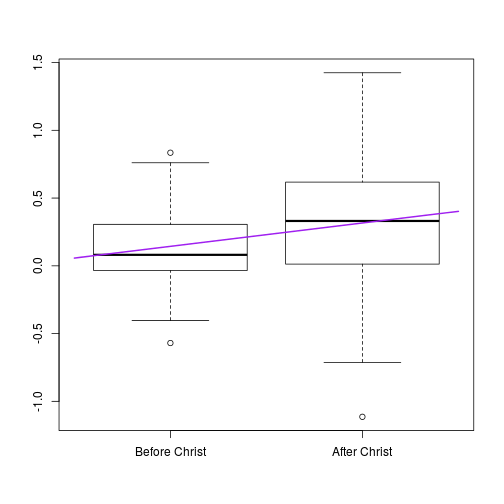## Well, that was a pain, but it shows an easy way to
## draw the confidence intervals.

newdf <- data.frame(xf = xlevels)
newdf$xf <- relevel(newdf$xf, ref = "Before Christ")
fit <- predict(m1, newdata = newdf, interval = "confidence")
newdf <- cbind(newdf, fit)

bp1 <-  boxplot(y ~ xf, data = dat)
lines(xnew, newdf$fit, col = "purple", lwd = 3) lines(xnew, newdf$lwr, col = 3, lwd = 2)
lines(xnew, newdf$upr, col = 3, lwd = 2)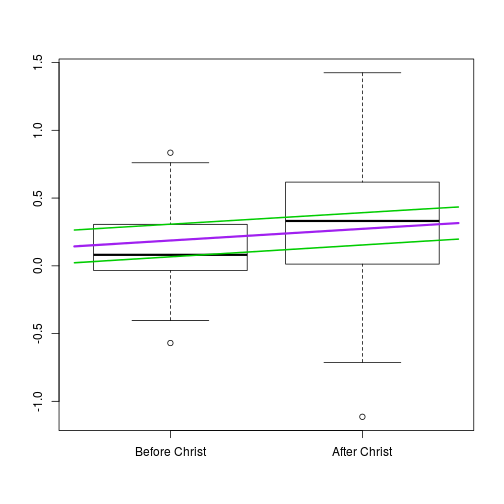## I said the "regression line" drawing is not my ## favorite for a dichotomous predictor. bp1 <- boxplot(y ~ xf, data = dat, border = gray(.8)) lines(c(x-0.5, x + 0.5), rep(y, 2), lwd = 2) lines(c(x-0.5, x + 0.5), rep(y, 2), lwd = 2) lines(c(x-0.25, x + 0.25), rep(newdf$lwr, 2),
col = 3, lwd = 1.5, lty = 2)
lines(c(x-0.25, x + 0.25), rep(newdf$lwr, 2), col = 3, lwd = 1.5, lty = 2) lines(c(x-0.25, x + 0.25), rep(newdf$upr, 2),
col = 3, lwd = 1.5, lty = 2)
lines(c(x-0.25, x + 0.25), rep(newdf$upr, 2), col = 3, lwd = 1.5, lty = 2)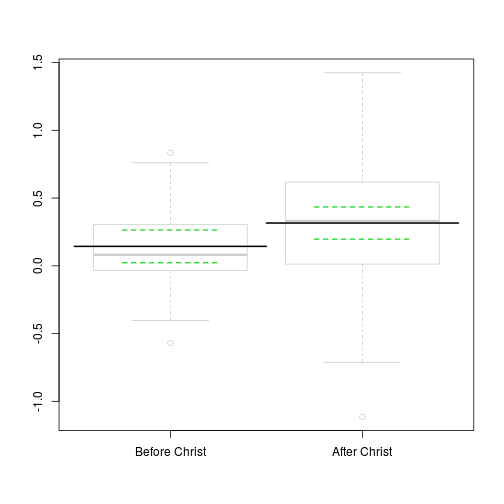## previous 4 commands are clear, but not concise. ## Can do better. Or assign students to. ## Vectorize it ## I'm not sure, but I think this is more beautiful with ## error bars, rather than just those boring lines. ## And lets make the predicted lines shorter bp1 <- boxplot(y ~ xf, data = dat, border = gray(.8)) lines(c(x-0.3, x + 0.3), rep(y, 2), lwd = 2) lines(c(x-0.3, x + 0.3), rep(y, 2), lwd = 2) s <- 1:2 arrows(as.numeric(newdf$xf[s]), newdf$lwr[s], as.numeric(newdf$xf[s]), newdf\$upr[s], col = "red", lty = 2, code = 3, angle = 90, length = .5, lwd = 1.5)